GeeksforGeeks App
Open AppBrowser
Continue

## Related Articles

• RD Sharma Class 10 Solutions

# Class 10 RD Sharma- Chapter 15 Areas Related to Circles – Exercise 15.3

### Question 1. AB is a chord of a circle with centre O and radius 4 cm. AB is of length 4 cm and divides the circle into two segments. Find the area of the minor segment.

Solution:

Given,

Radius of the circle with centre O, given by OA and OB = r = 4 cm

Also, length of the given chord AB = 4 cm

Since all the sides are equal, OAB is an equilateral triangle where the angle AOB = 60°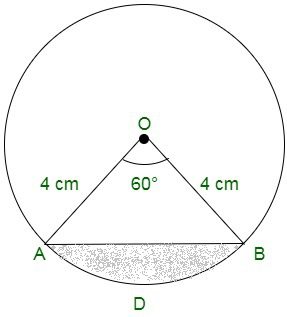Thus, the angle subtended at the centre θ is equal to 60°

Now,

Area of the minor segment = (Area of sector) – (Area of triangle AOB)Therefore, now the required area of the segment is given by (8π/3 – 4√3) cm2

### Question 2. A chord PQ of length 12 cm subtends an angle 120o at the centre of a circle. Find the area of the minor segment cut off by the chord PQ.

Solution:

We have, ∠POQ = 120o and the line segment PQ = 12 cm

Draw the line segment OV ⊥ PQ,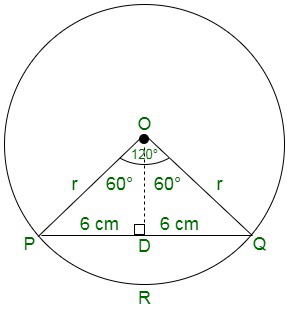Now,

PV = PQ × (0.5) = 12 × 0.5 = 6 cm

Since, ∠POV = 120o

Also,

∠POV = ∠QOV = 60o

We have,

In triangle OPQ, we have

Sin θ = PV/ OA

Sin 60o = 6/ OA

Substituting the values, we get,

√3/2 = 6/ OA

OA = 12/ √3 = 4√3 = r

Now, calculating the area of the minor segment

Area of the segment = area of sector OPUQO – area of △OPQ

= θ/360 x πr2 – ½ x PQ x OV

= 120/360 x π(4√3)2 – ½ x 12 x 2√3

= 16π – 12√3 = 4(4π – 3√3)

Hence,

Area of the minor segment = 4(4π – 3√3) cm2

### Question 3. A chord of a circle of radius 14 cm makes a right angle at the centre. Find the areas of the minor and major segments of the circle.

Solution:

Given,

Radius (r) of the circle is given by = 14 cm

Angle subtended by the chord with the centre of the circle, θ is given by 90°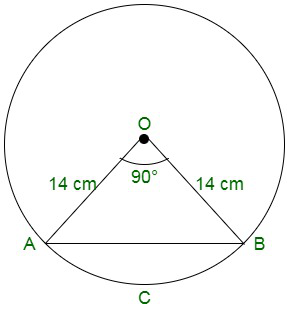Now,

Area of minor segment = θ/360 x πr2 – ½ x r2 sin θ

Substituting the values, we get,

= 90/360 x π(14)2 – ½ x 142 sin (90°)

= ¼ x (22/7) (14)2 – 7 × 14

= 56 cm2

Area of circle = πr2

Substituting the values,

= 22/7 x (14)2 = 616 cm2

Thus, we know,

The area of the major segment = Area of circle – Area of the minor segment

= 616 – 56 = 560 cm2

### Question 4. A chord 10 cm long is drawn in a circle whose radius is 5√2 cm. Find the area of both the segments.

Solution:

Given,

Radius of the circle, r = 5√2 cm = OA = OB

Length of the chord AB = 10 cm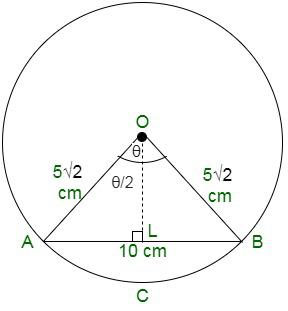Now,

In triangle OAB,AB = 100

Thus, Pythagoras theorem is satisfied, that is, OAB is a right-angled triangle.

Angle subtended by the chord at the centre of the circle, θ = 90°

We know,

Area of minor segment = area of sector – area of triangle

Substituting the values,

= θ/360 × πr2 – ½ x r2 sin θ

= 90/360 × (3.14) 5√22 – ½ x (5√2)2 sin 90

= [¼ x 3.14 x 25 x 2] – [½ x 25 x 2 x 1]

We obtain,

= 25(1.57 – 1)

= 14.25 cm2

Area of circle = πr2 = 3.14 x (5√2)2 = 3.14 x 50 = 157 cm2

Thus,

Area of major segment = Area of circle – Area of minor segment

= 157 – 14.25

= 142.75 cm2

### Question 5. A chord AB of circle of radius 14 cm makes an angle of 60° at the centre of a circle. Find the area of the minor segment of the circle.

Solution:

Given,

Radius of the circle (r) is given by= 14 cm = OA = OB

Angle subtended by the chord at centre of the circle, θ = 60°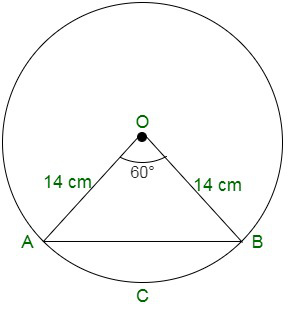In triangle AOB, since, angle opposite to equal sides are equal,

Therefore, angle A = angle B

By angle sum property,

∠A + ∠B + ∠O = 180

x + x + 60° = 180°

2x = 120°, x = 60°

Since, all the angles are 60° each, therefore, the triangle OAB is an equilateral with OA = OB = AB

We know,

Area of the minor segment = area of sector – area of triangle OAB

= θ/360 × πr2 – 1/2 r2 sin θ

= θ/360 × πr2 – 1/2 x (14)2 sin 60°

= 60/360 × (22/7) 142 – 1/2 x (14)2 x √3/2

= 60/360 x (22/7) 142 – √3/4 x (14)2

= 142 [(1/6) x (22/7)] – 0.4330

= 142 [(22/42)] – 0.4330

= 142 [(11-9.093)/21]

= 142 [0.09080]

= 17.79

Therefore, the area of the minor segment = 17.79 cm2

### Question 6. Find the area of the minor segment of a circle of radius 14 cm, when the angle of the corresponding sector is 60°. [Ncert Exemplar]

Solution:

We have,

Radius of the circle, r = 14 cm

Central angle of the corresponding sector = 60°

Since, OA = OB = r, therefore, triangle AOB is an isosceles triangle.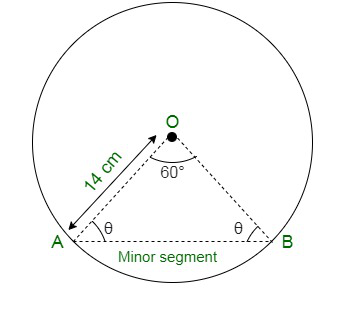∠OAB = ∠OBA =  θ

Now, in triangle OAB, by angle sum property

∠AOB + ∠OAB + ∠OBA = 180°

Substituting values,

60° +  θ + θ = 180°

Solving,

θ = 60°

Now, since all the angles are equal , therefore it is an equilateral triangle, with equal sides, that is,

OA = OB = AB = 14 cm

Area of triangle OAB =Substituting the values, we get

Area = 49 √3 cm2

Also,

Area of sector OBAO =Now,

Area of minor segment = Area of sector OBAO – Area of triangle OAB =### Question 7. A chord of a circle of radius 20 cm subtends an angle of 90° at the centre. Find the area of the corresponding major segment of the circle. (Use π = 3.14) [Ncert Exemplar]

Solution:

Let AB be the chord of a circle of radius 10 cm, with O as the centre of the circle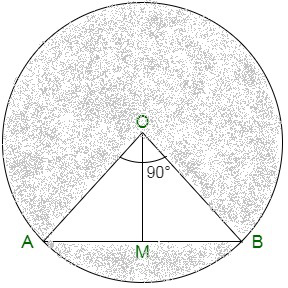We know,

∠AOB = 90°

Therefore,

Angle of the major sector = 360° – 90° = 270°

Therefore,

Area of the major sector =Now,

Draw OM perpendicular to AB

So,

AM = 1/2 AB and ∠AOM = 1/2×90° = 45°

Now,So,

AM = 10/√2

Therefore, AB = 10√2 cm and also, OM=OASo,

Area of triangle OAB = 1/2 * base * heightArea of the required major segment = 235.5 cm2 + 50 cm2 = 285.5 cm2

Also,

Area of triangle OAB = 1/2 × OA × OB

= 1/2 × 10 × 10 cm2 = 50 cm2

### Question 8. The radius of a circle with centre O is 5 cm (see figure). Two radii OA and OB are drawn at right angles to each other. Find the areas of the segments made by the chord AB (Take π = 3.14).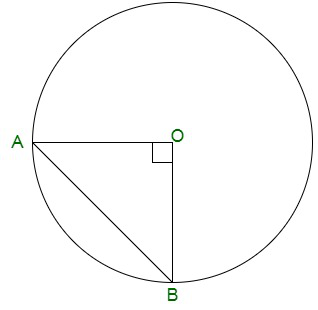Solution:

Radius of the circle ,r = 5 cm

Since, OA and OB are at right angle

Therefore, ∠AOB = 90°

∵  Chord AB makes two segments which are minor segment and major segment respectively.

Now,

Area of the minor segmentArea of circle = pi * r2 = 78.50 cm2

Therefore, area of major segment = 71.375 sq. units.

### Question 9. AB is the diameter of a circle, centre O. C is a point on the circumference such that ∠COB = 0. The area of the minor segment cut off by AC is equal to twice the area of the sector BOC. Prove that.Solution: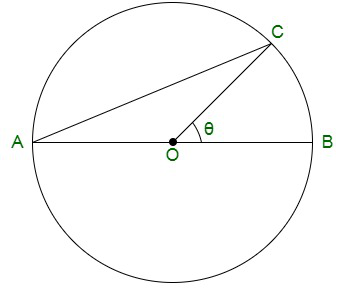Given,

∠AOC = 180° – θ

Let us assume r to be the radius of the circle.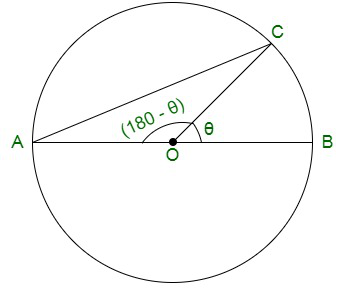Area of sector BOC =Now,

Area of minor segment AC = 2 * Area of sector BOCBut, Area of segment=Now,Also,### Question 10. A chord of a circle subtends an angle of 0 at the centre of the circle. The area of the minor segment cut off by the chord is one eighth of the area of the circle. Prove thatSolution:

Let chord AB subtends an angle of 0° at the centre of a circle with radius r

Now area of the circle = nr1 and area of the minor segment ACB.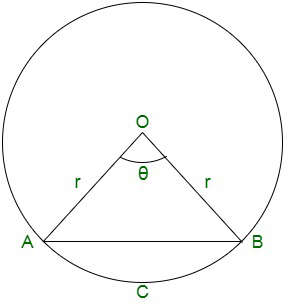But.

Area of the minor segment ACB = 1/8 = area of circleMy Personal Notes arrow_drop_up
Related Tutorials